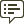Community Profile### MathWorks

Last seen: Today Active since 2014

Professional Interests:

#### Statistics

All
••••••••••••#### Content Feed

View by

Creating a polar bar chart or circumplex chart (not a histogram)
See polarhistogram. If you have data suitable for a bar plot and, therefore, you don't need to compute the bins and bar heights...

4 días ago | 2

| accepted

Take 102 Elements Equally Spaced of an Existing Array
> Is there a way to solve this issue? No. 991 is not divisible by 102. The following is a 102 vector starting with 1 and end...

4 días ago | 1

| accepted

Readtable don't work correctly if 'Range' > 'A250'
That doesn't sound good. If you manually grab the import options, that addresses the problem. opts = spreadsheetImportOptio...

5 días ago | 0

| accepted

how can I plot a graph over an image in Matlab?
Plot the image using image or imagesc or some other image function that allows you to specify the x and y values of the image. ...

6 días ago | 2

Accessing cell array contents
See cellfun In this example, the cell array is named myCellArray and the variable is named myVar. y = cellfun(@(A)A.myVar(en...

6 días ago | 2

Converting wind/wave data to hourly data by interpolation
If the durations are consecutive, then I suggest creating an time stamps based on the durations which allows you to use retime t...

6 días ago | 0

| accepted

How to plot correlation coefficient matrix plot?
Assuming you already have the correlation matrix, use heatmap. The upper triangle can be filled with NaNs. S = load('Data_Can...

6 días ago | 1

A green arrow in an m-file
You are in debug mode. The green arrows hows the line at which MATLAB is currently paused. how did I get here? There a...

7 días ago | 0

| accepted

+60k Lines on one plot - Too slow
Another idea is to plot your 60k lines as a 2D density plot (initially suggested in this thread). Pros: 1 graphics object, l...

10 días ago | 0

| accepted

How to convert fprintf from single row to multiple rows?
I assume you want to show each numeric value in a new line. To do that, add a new line control character where you want to inse...

10 días ago | 0

how I show all the numbers in y axes
> its important to me that all the numbers appears even these small changes Is your concern that readers will not believe that ...

11 días ago | 0

Sorting table row variables with number and letters
Use TableC = sortrows(TableB,'RowNames') This option also appears in the OP's 2017b documentation.

11 días ago | 1

| accepted

What does a(a<0) = 0 mean in matlab?
> What does a(a<0) = 0 mean? a can be a scalar or an array. This replaces all values in a that are less than 0 with 0. Ex...

13 días ago | 1

Box plot into figure with another graphs
MATLAB's boxplot and boxchart are designed to create box plots from the raw data. boxplot(Predictions_sorted) % or boxchar...

13 días ago | 0

| accepted

Standard deviation in 3D array from 1st to nth number array
To cumulatively compute the std across dimension 3, data = rand(6, 493, 6000); RN = zeros(size(data,[1,3])); for i = 1 : si...

13 días ago | 0

"Graphics timeout occurred"
Can you reproduce this issue? If so, sharing the steps that reproduce this would be helpful. Nevertheless, please report thi...

15 días ago | 0

| accepted

how to compare column values of a matrix with a column vector of different size?
Load data detected = [56 40 33 31 28 13 10 1]; M_transmision = [1 40 -84 -638 6...

15 días ago | 0

| accepted

Convert double to categorical array
The function categorical can convert continuous data into categorical data. However, this function cannot categorize two numbers...

19 días ago | 0

| accepted

'Value' must be double scalar within the range of 'Limits'

19 días ago | 1

| accepted

Best way to analyze two waveform signals
Hello @Austin Bollinger, looks like @Jonas has made some good recommendations. I'll add another perspective. My grad school r...

19 días ago | 0

| accepted

Creating callable function with prompts
txt = input(prompt,"s") Include the "S" in argument 2 to return the entered text without evaluating it. > created a bunch o...

19 días ago | 0

Set xaxis of imagesc with scaled values
Specify x and y values using imagesc(x,y,C) I think this is what you're looking for C = rand(20,400); % Replace this with your...

2 meses ago | 0

| accepted

change focus in app desinger
> Is there a way to put the cursor in the editbox without click the box Yes, as of MATLAB R2022a. See the focus function whic...

2 meses ago | 0

Why is my p-value matrix after using corrcoef filled with NaN?
First check whether you have NaNs in your inputs. If so, that explains why there are NaNs in your outputs. If you do not have...

2 meses ago | 0

| accepted

Missing legend entries in plotyy
Use yyaxis instead of plotyy. Instead of using plotyy(x1,y1,x2,y2) use yyaxis left plot(x1,y1) yyaxis right plot(x2,y2...

2 meses ago | 0

How to pick maximum value in group?
data = [0.74 0.66 0.58 0.47 0.606 0.705 0.76]; group = [6 6 5 6 5 6 6]; groupsummary(data(:),group(:),'max')...

3 meses ago | 0

| accepted

Using for loop to find values greater than a number in each column.
It's a pitty that the homework assignment requires using a loop since this can be solved in one line of code. y = mat > mean(m...

3 meses ago | 0

Highlighted

National Volunteer Week: Contribute to the MATLAB Central Community
<<https://www.mathworks.com/matlabcentral/discussions/uploaded_files/11465/data>>  *20 minutes makes a difference* I...

3 meses ago | 13